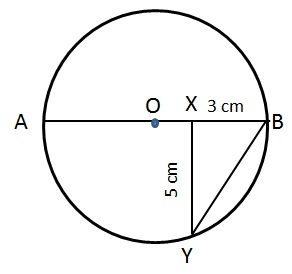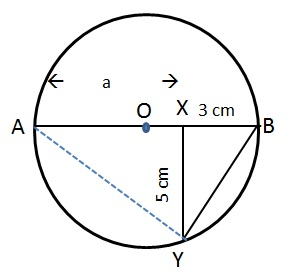# CAT Quantitative Aptitude Questions | Geometry Questions for CAT - Triangles

###### CAT Questions | CAT Geometry Questions | Circles

The question is from the topic circles. From the diagram, we have to find out the circumference of the circle. A perpendicular to the diagram of the circle and it's distance is given. CAT Geometry questions are heavily tested in CAT exam. Make sure you master Geometry problems.

Question 31: What is the circumference of the below circle given that AB is the diameter and XY is perpendicular to AB?1. 8π cm
2. π√34 cm
3. 34π/3 cm
4. π√31/3 cm

## Best CAT Coaching in Chennai

#### CAT Coaching in Chennai - CAT 2022Limited Seats Available - Register Now!

##### Method of solving this CAT Question from Circles: Finding the radius of the circle will help! Look at the triangles present!.Join AY and let AX = a
Diameter = a + 3
Since AB is the diameter, ∠AYB = 90
Since XY is perpendicular to AB, ∠BXY = 90
BY = √(32+52) = √34
Consider triangles ABY and BXY
∠ABY is same as ∠XBY and both the triangles are right angled
Therefore they are similar triangles and sides are proportional!
AB/BY = BY/BX = AY/XY
(a+3)/√34 = √34/3
3a + 9 = 34
or a = 25/3
Diameter = 25/3 + 3 = 34/3
Circumference = π * d = 34π/3 cm

The question is "What is the circumference of the below circle given that AB is the diameter and XY is perpendicular to AB?"

##### Hence, the answer is 34π/3 cm.

Choice C is the correct answer.

###### CAT Coaching in ChennaiCAT 2023

Classroom Batches Starting Now! @Gopalapuram

###### Best CAT Coaching in Chennai Introductory offer of 5000/-

Attend a Demo Class

## CAT Preparation Online | CAT Geometry questions Videos On YouTube

#### Other useful sources for Geometry Question | Geometry Triangles Circles Quadrilaterals Sample Questions

##### Where is 2IIM located?

2IIM Online CAT Coaching
A Fermat Education Initiative,
58/16, Indira Gandhi Street,
Kaveri Rangan Nagar, Saligramam, Chennai 600 093

##### How to reach 2IIM?

Phone: (91) 44 4505 8484
Mobile: (91) 99626 48484
WhatsApp: WhatsApp Now
Email: prep@2iim.com